# Spring Constant Formula

Spring Constant Formula

Springs have their own natural "spring constants" that define how stiff they are. The letter k is used for the spring constant, and it has the units N/m. By Newton's Third Law of Motion, as a spring is pulled, it pulls back with a restoring force. This force follows Hooke's Law, which relates the force of the spring to the spring constant, and the displacement of the spring from its original position.

force of the spring = -(spring constant k)(displacement)

F = -kx

F = restoring force of the spring (directed toward equilibrium)

k = spring constant (units N/m)

x = displacement of the spring from its equilibrium position

Spring Constant Formula Questions:

1) Find the spring constant of a spring if it requires a 9000 N force to pull it 30.0 cm from equilibrium.

Answer: The formula can be rearranged to solve for the spring constant, k: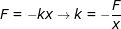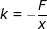In this question, a 9000 N force is pulling on a spring. That means that the spring pulls back with an equal and opposite force of -9000 N. The displacement is 30.0 cm = 0.30 m.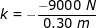k = 30 000 N/m

The spring constant of this spring is 30 000 N/m.

2) A 3500 N force is applied to a spring that has a spring constant of k = 14 000 N/m. How far from equilibrium will the spring be displaced?

Answer: The displacement can be found by rearranging the formula:In this question, a 3500 N force is pulling on a spring. That means that the spring pulls back with an equal and opposite force of -3500 N.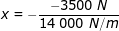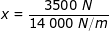x = 0.250 m

x = 25.0 cm

The spring is displaced by 0.250 m, which is equal to 25.0 cm.

 Related Links: Potential Energy: Elastic Formula